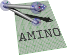amino Lightweight Robot Utility Library
Class List
Here are the classes, structs, unions and interfaces with brief descriptions:
[detail level 123]
 ▼Namino Amino namespace CAxisAngle An axis-angle object CDualQuat A dual quaternion object CQuat A quaternion object CQuatTran A rotation quaternion and translation vector object ▼CRegionAllocator An STL allocator that allocates out of a memory region Crebind CRegionList Typedefs for STL lists using region allocator CRegionMap Typedefs for STL map using region allocator CRegionVector Typedefs for STL vector using region allocator CRotMat A rotation matrix object CScopedMutex Locks mutex on construction, unlocks on destruction CTfMat A transformation matrix object CVec3 A vector of length 3 CXAngle A rotation about the X axis CYAngle A rotation about the Y axis CZAngle A rotation about the Z axis Caa_circbuf_t Circular buffers use a fixed-size array that acts as if connected end-to end Caa_flexbuf_t A buffer struct Caa_mem_cons A "cons" cell Caa_mem_pool_t Data Structure for Object pools Caa_mem_region Data Structure for Region-Based memory allocation Caa_mem_region_node A single block of memory to be parceled out by the region allocator Caa_mem_rlist A linked list allocated out of a memory region Caa_ode_sol_opts Options for the differential equation solver Caa_plot_opts Options for a plot Caa_rx_geom Container for scene geometry Caa_rx_sg Opaque type for a scene_graph Caa_rx_shape_box Shape for a box Caa_rx_shape_cone Shape for a cone Caa_rx_shape_cylinder Shape for a cylinder Caa_rx_shape_grid Shape for a grid Caa_rx_shape_sphere Shape for a sphere Caa_rx_win Opaque type for a window Caa_sdl_display_params Parameters for SDL display function Caa_sys_affine_t Context-struct for function aa_sys_affine Caa_tf_axang Axis-Angle rotation Caa_tf_duqu Memory layout for a dual quaternion Caa_tf_dx Memory layout for an SE(3) velocity Caa_tf_eulerzyx Memory layout for an Euler Angle in ZYX (yaw-pitch-roll) format Caa_tf_quat Memory layout for a quaternion, x,y,z,w order Caa_tf_qv Memory layout for a Transformation as rotation quaternion and translation vector Caa_tf_qv_dx Transform and spatial velocity Caa_tf_rotmat Memory layout for a rotation matrix Caa_tf_tfmat Memory layout for a transformation matrix Caa_tf_vec3 Memory layout for a vector of length 3 CMat CTf CVec